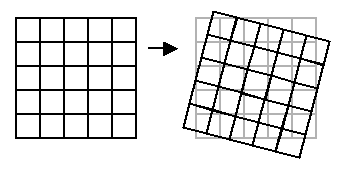# Pixel interpolation

In transforming an image geometrically, for example rotating it or applying a gnomonic projection, it often happens that integral (whole-number) coordinates x and y for the source pixel become non-integral destination coordinates.For an accurate transformation it is therefore necessary to spread the brightness of the transformed pixel over several adjacent pixels, proportionately to the amount of overlap with each. This is not very feasible however, because there is no guarantee that all pixels in the destination image will receive values, especially if the transformation involves stretching. We would end up with holes in the image.

A simple way of avoiding the holes is to work backwards. In other words calculate the inverse transformation. For each pixel in the destination image work out where it should have come from. The coordinates of the destination point will then be whole numbers but the source coordinates will not be. But then it is simpler to sample proportionately from neighbouring pixels.

If the non-integral sampling point is (x, y) the sampled value should be

fx . [fy . p (tx + 1, ty + 1) + (1 - fy) . p (tx + 1, ty)] +
(1 - fx) . [fy . p (tx, ty + 1) + (1 - fy) . p (tx, ty)]

where fx & fy denote the fractional parts of x, y and
tx & ty denote the truncated (whole number) parts.

This is implemented in net.grelf.Interpolator.getPixel () and also in the implementations of net.grelf.grip.Image.getPixelInterpolated (). Higher level operations such as net.grelf.image.Image.rotate () then use those methods.

Next page